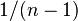# Difference between revisions of "Multiple-choice question"

## Definition

A multiple-choice question' (MCQ) is a question where multiple choices (also called options) are provided, and the respondent is expected to select one or more of the options based on the question.

Multiple-choice questions could be:

• questions where respondents are expected to pick a single response
• questions where respondents are expected to pick one or more options in response

Further, they could involve:

• a pre-specified correct answer option (or option set) against which the respondent's option is compared
• a survey multiple-choice question, that is intended to get information about the respondent's preference or individual-specific information. In this case, there is no right answer.

This page is not about survey MCQs. Rather, it is about questions where there is a single specific correct answer. For clarity, we will restrict attention to the case where respondents are expected to pick a single response. Cases where respondents need to pick sets of options can be reduced to this case through a mathematical equivalence.

## Related concepts

### Controlling for random guesses: the basic model

Here, we assume no scoring penalty for random guesses, and we assume that respondents attempt every question.

The simplest model for controlling for random guesses is that for every question, every respondent falls into one of two categories:

• The respondent knows the correct answer
• The respondent makes a guess that has a$1/n$ probability of being correct where$n$ is the number of options

If we follow this model, then:

Expected number of answers the respondent does not know =$n/(n - 1)$ * (Apparent number of wrong answers)

Or equivalently:

Expected number of answers the respondent knows = Number of answers the respondent gets right -$1/(n - 1)$ * (Number of answers the respondent get wrong)

Similar formulas apply to the study of a single question across multiple respondents.

The basic model is unrealistic for a number of reasons, namely that people often have specific misconceptions or make specific careless errors that lead them to select wrong answers. On the other hand, some might guess the right answer with a higher probability than mere chance through a process of elimination, without actually knowing the answer. The design of the options is extremely relevant to inferring the respondent's knowledge from the responses.

### MCQs that have clear answers independent of seeing the options versus MCQs whose answer depends on the options

In some cases, the MCQ is a clear question with a clear, objective answer that can be determined without looking at the options. For instance, a question like "What number is 2 + 2?" has a clear answer that one can work out independently -- and then check against the options to see which one it matches.

On the other hand, a MCQ of the form "Which of the following statements about mammals is true?" requires an examination of all the available options. It would not be possible to generate the answer by merely looking at the question and then compare it against the options.

### The use of free responses to generate options

Some versions of test design, particularly for concept inventories, use free responses to generate the options for the multiple choice questions. Explicitly, students are asked to write down answers to the question part of the MCQ in free response form. Some form of text analysis is done on the answers to extract the most frequent lines of student thought, and these are used to formulate the incorrect answer options. Note that such a process is highly likely to generate good distractors (see below) as the incorrect answer options.

### Distractors

A distractor is defined as an option for a multiple-choice question that is incorrect, but that can be arrived at as the correct answer by a respondent who has a particular misconception or makes a particular kind of error in reading or working out the question, where the misconception or error are relatively likely. The way that distractors are used is a major part of the design of the MCQ, and considerably affects its perceived difficulty.

In general, a distractor should be chosen such that the distinction between the correct answer and the distractor is part of the goal of the assessment.

For instance, suppose solving a problem requires a conjunction of skills A and B. Some people make careless errors with the part that requires skill B, leading them to converge on a particular wrong answer. If testing the error-free application of skill B is part of our goal, it makes sense to include the incorrect answer as a distractor. If, however, the primary goal is to test skill A, then the distractor does not make sense.

Distractors can also be used for diagnostic purposes. For instance, if answering a question requires a conjunctoin of skills, and erroneous application at each level leads to a particular distractor, and these distractors are separate, choosing the different distractors as options makes it possible to perform an analysis of responses and determine what misconceptions dominated. The use of multiple distractors per question, however, may depress the total score of individuals too much and be bad for assessments based on total scores.